Saturday , July 31 2021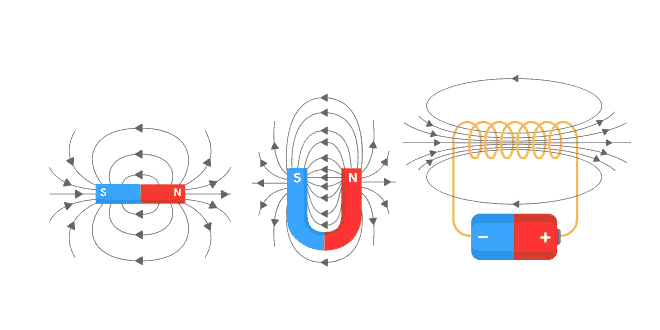# Magnetic Effects of Electric Current: 10 Science

#### Question: In our daily life we use two types of electric current whose current-time graphs are given below: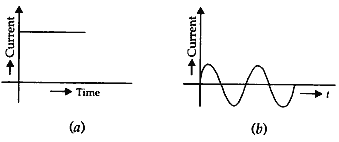#### (1). Name the type of current in two cases. (2). Identify any one source for each type of current. (3). What is the frequency of current in case (b) in our country? (4). On the basis of these graphs list two differences between the two currents. (5). Out of the which one is used in transmitting electric power over long distances and why?

1. (a) Direct current  (b) Alternating current.
2. Source of direct current: battery. Source of alternating current: ac generator.
3. Frequency of ac in our country is 50 Hz.
4. Magnitude of current in dc remains fixed while in ac it changes continuously. Direction of current in dc remains fixed while in ac it changes continuously. Direction of current in dc remain fixed while is ac it changes after every 1/2n second, where n is the frequency of ac.
5. For transmitting electric power over long distances ac is used because loss of energy during transmission is comparatively very small as compared to that of dc.

#### Question: (a) Describe an experiment with a diagram to show that force is exerted on a current carrying conductor when placed perpendicular in a magnetic field. (b) How will this force change if current in the conductor is increased? (c) Name a device that uses the above principle.

Answer: (a) Consider the rod shown in the figure which is placed in a magnetic field. When current is passed through the rod, it gets deflected. This shows that a current carrying conductor in a magnetic field experiences a force.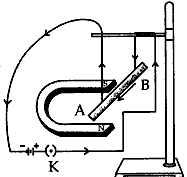(b) The force acting on the rod will the rod will increase. This will increase the displacement of the rod.

(c) An electric motor.

#### Question: (a) Which effect of the electric current is utilised in the working of an electrical fuse? (b) A fuse is connected in series or in parallel in household circuit? (c) Draw a schematic labelled diagram of a domestic circuit which has a provision of a main fuse, meter, one light bulb and a switch and a switch socket.

(b) Series.
(c) The diagram is as shown below: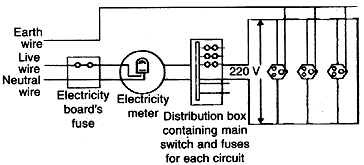#### Question: (1). Draw a schematic diagram showing common domestic circuit. (2). State the function of an earth wire. Why is it necessary to earth metallic appliances such as an electric iron?

1. See Q.23 Part (c).
2. Function of an earth wire: The metallic body the electric appliances is connected to the earth by means of earth wire so that any leakage of electric current is transferred to the ground. Earthing of metallic appliances prevents the user from electric shocks in case of any leakage.

#### Question: (a) Define electromagnetic induction. (b) Two coils P and S are would over the same iron core. Coil P is connected to battery and key and the coil S is connected to galvanometer. Draw a suitable diagram of this arrangement and write your observations when: (i) Current in the coil P is started by closing the key. (ii) Current continues to flow in coil P. (iii) Current in coil P is stopped by removing the key. Explain the reason for such observations.

Answer: (a) It is defined as the phenomenon by which a changing magnetic field in a conductor induces a current in another conductor.

(b). The diagram is as shown: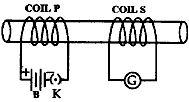(i) There is deflection in galvanometer connected with coil due to induced current. This is due to the changing magnetic field which induced current in the coil.
(ii) Deflection becomes zero. Since current become constant, therefore, there will be no change in magnetic field, hence no induced current.
(iii) Deflection in galvanometer is in opposite direction. Induction takes place but induced current is in opposite direction due to the current decreasing to zero.

#### Question: Two coils C1 and C2 are wrapped around a non-conducting cylinder. Coil C1 is connected to a battery and key and C2 with galvanometer G. On pressing the key (k), current starts flowing in the coil C1. State your observation in the galvanometer.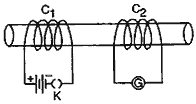#### (i) When key K is pressed on. (ii) When current the coil C1 is switched off. (iii) When the current is passed continuously through coil C1. (iv) Name and state the phenomenon responsible for the above observation. Write the name of the rule that is used to determine the direction of a current produced in the phenomena.

Answer: (i) Galvanometer will show momentary deflection towards one side.
(ii) Galvanometer will show momentary deflection but in direction opposite to the previous one.
(iii) Galvanometer will not show any deflection.
(iv) The phenomenon is electromagnetic induction. It’s a process in which a changing magnetic field in a conductor induces a current in another conductor near it.

Fleming’s right hand rule is used to find the direction of current.

####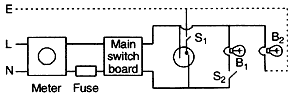(a) Fuse is incorrectly connected the neutral wire (N), It must be connected to the live wire (L).
(b) Bulb B2 is not connected to the neutral wire.

(ii) Element of an electric heater – high melting point. element of a fuse – low melting point.

(iii) (a) Each appliance has equal potential difference.
(b) Each appliance has separate switch to ON/OFF the flow of current through it.

#### Question: Why does a compass needle gets deflected when brought near a bar magnet?

Answer: A compass needle is, in fact, a small bar magnet. If this is brought near another bar magnet, the like poles repel and the needle gets deflected.

#### Question: List the properties of magnetic lines of force.

a) Magnetic lines are directed from the north pole towards the south pole.
b) They do not cross each other.
c) They are more crowded near the poles than at any other region in the field.
d) They are closed curves.
e) In the uniform magnetic field, the lines of force are parallel to one another.

#### Question: Why don’t two magnetic lines of force intersect each other?

Answer: No two field-lines are found to cross each other. If they did, it would mean that at the point of intersection, the compass needle would point towards two directions, which is not possible.

#### Question: Consider a circular loop of wire lying in the plane of the table. Let the current pass through the loop clockwise. Apply the right-hand rule to find out the magnetic field inside and outside the loop.

Answer: At every point of a current –carrying loop, the concentric circles representing the magnetic field around it would become larger and larger as we move away from the wire. By the time we reach at the center of the circular loop, the arc of these big circles would appear as straight lines.

#### Question: The magnetic field in a given region is uniform. Draw a diagram to represent it.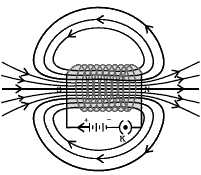#### Question: (a) Current in rod AB is increased? (b) A stronger horseshoe magnet is used? (c) Length of the rod AB is increased?

(a) If the current in rod AB is increased, the displacement of rod AB will not be affected.
(b) If a stronger horseshoe magnet is used, force is exerted and hence the displacement increases.
(c) If the length of the rod AB is increased there is no change in the displacement of the rod AB.

#### Question: State Fleming’s left-hand rule.

Answer: Fleming’s left-hand rule states that, stretch the thumb, fore finger and middle finger of the left hand such that they are mutually perpendicular. If the first finger points in the direction of magnetic field and the second finger in the direction of current, then the thumb will point in the direction of motion or the force acting on the conductor.

#### Question: What is the principle of an electric motor?

Answer: Principle of an electric motor: The working of the electric motor is based on the mechanical effect of an electric current. A conductor carrying a current placed in a magnetic field experiences a mechanical force.
In the motor, when a current is passed through a rectangular coil of wire placed in a magnetic field, the coil rotates continuously.

#### Question: What is the role of the split ring in an electric motor?

Answer: In electric motor, the split ring acts as a commutator. A device that reverses the direction of flow of current through a circuit is called a commutator. The reversal of current also reverses the direction of force acting on the two arms AB and CD.

#### Question: Explain different ways to induce current in a coil.

Answer: Current can be induced in a coil either by moving it in a magnetic field or by changing the magnetic field around it. The induced current is found to be the highest when the direction of motion of the coil is at right angles to the magnetic field. The process, by which a changing magnetic field in a conductor induces a current in another conductor, is called electromagnetic induction.

#### Question: State the principle of an electric generator.

Answer: A generator is also known as a dynamo. It is a device used to convert mechanical energy in to electrical energy. The mechanical energy is used to rotate a conductor in a magnetic field to produce electricity. It is an application of electromagnetic induction.
An A.C generator generates an alternating current.
A D.C generator is used to deliver a current, which flows in the same direction.

#### Question: Name some source of direct current.

Answer: The source of direct current is a split-ring type commutator, one brush is at all times in contact with the arm moving up in the field, while the other is in contact with the arm moving down. Thus a unidirectional current is produced.

#### Question: Which sources produce alternating current?

Answer: The sources which produce alternating current is a permanent magnet called the field magnet, armature, slip ring and carbon brushes. After every half rotation the polarity of the current in the respective arms changes. Such a current, Which changes direction after equal intervals of time, is called an alternating current.

## 10th CBSE Science Pre Board Exam 2020-21

10th CBSE Science Pre Board Exam 2020-21 School Name: Himalaya Public School, Sector 13, Rohini, Delhi 110085 India Time: 3 …